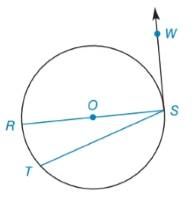Chapter 6.2, Problem 3E### Elementary Geometry for College St...

6th Edition
Daniel C. Alexander + 1 other
ISBN: 9781285195698

#### Solutions

Chapter
Section### Elementary Geometry for College St...

6th Edition
Daniel C. Alexander + 1 other
ISBN: 9781285195698
Textbook Problem
3 views

# Given: Circle O with diameter R S ¯ , tangent S W → , chord T S ¯ and m R T ⌢ = 26 ° Find: a ) m ∠ W S R b ) m ∠ R S T c ) m ∠ W S TTo determine

(a)

To find:

mWSR where circle O with diameter RS¯, tangent SW, chord TS¯ and mRT=26°.

Explanation

Theorem:

The radius drawn to a tangent at the point of tangency is perpendicular to the tangent at that point.

Calculation:

To find: mWSR.

To determine

(b)

To find:

mRST when mRT=26°.

To determine

(c)

To find:

mWST.

### Still sussing out bartleby?

Check out a sample textbook solution.

See a sample solution

#### The Solution to Your Study Problems

Bartleby provides explanations to thousands of textbook problems written by our experts, many with advanced degrees!

Get Started

#### (3, 6)

Applied Calculus for the Managerial, Life, and Social Sciences: A Brief Approach

#### Find dy/dx by implicit differentiation. 7. x4 + x2y2 + y3 = 5

Single Variable Calculus: Early Transcendentals, Volume I

#### Solve the equations in Exercises 126. (x21)2(x+2)3(x21)3(x+2)2=0

Finite Mathematics and Applied Calculus (MindTap Course List)

#### What are the two requirements for a random sample?

Essentials of Statistics for The Behavioral Sciences (MindTap Course List)

#### True or False: is a convergent series.

Study Guide for Stewart's Multivariable Calculus, 8th